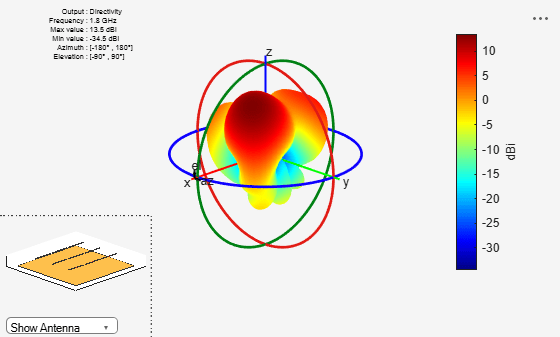Documentation

# phaseShift

Calculate phase shift values for arrays or multi-feed PCB stack

## Syntax

``ps = phaseShift(array,frequency,angle)``
``ps = phaseShift(pcb,frequency,angle)``

## Description

example

````ps = phaseShift(array,frequency,angle)` calculates the phase shift values of an array operating at a specified frequency to scan the beam at the given angle. The velocity of light is assumed to be that in free space.```
````ps = phaseShift(pcb,frequency,angle)` calculates the phase shift values of a multi-feed PCB stack at a specified frequency and angle.```

## Examples

collapse all

Create a 3-by-3 rectangular array of reflector-backed dipoles at an operating frequency of 1.8 GHz, and scan the main beam at 30 degrees along the azimuth and 45 degrees along the elevation.

```a = design(rectangularArray('Size',[3 3]),1.8e9,reflector); ps = phaseShift(a,1.8e9,[30;45])```
```ps = 9×1 350.5337 54.1733 117.8129 240.3066 303.9462 7.5858 130.0796 193.7192 257.3588 ```
`a.PhaseShift = ps`
```a = rectangularArray with properties: Element: [1×1 reflector] Size: [3 3] RowSpacing: 0.0833 ColumnSpacing: 0.0833 Lattice: 'Rectangular' AmplitudeTaper: 1 PhaseShift: [9×1 double] Tilt: 0 TiltAxis: [1 0 0] ```

Calculate the radiation pattern of the array.

`pattern(a,1.8e9)`## Input Arguments

collapse all

Antenna array from the Antenna Toolbox™ array library, specified as an array object.

Example: ```r = rectangularArray; phaseShift (r,70e6,[60;40])```. Calculates the phase shift of the rectangular array.

Multi-feed PCB stack, specified as a `pcbStack` object.

Example:

Frequency value used to calculate the phase shift, specified as a scalar in Hz.

Example: `70e6`

Data Types: `double`

Azimuth and elevation angle pair to scan the array toward, specified as a 2-element vector in degrees.

Example: `[35;40]`

Data Types: `double`

## Output Arguments

collapse all

Phase shift values, returned as a 1-by-N vector in degrees. Phase shift value calculation does not consider mutual coupling.